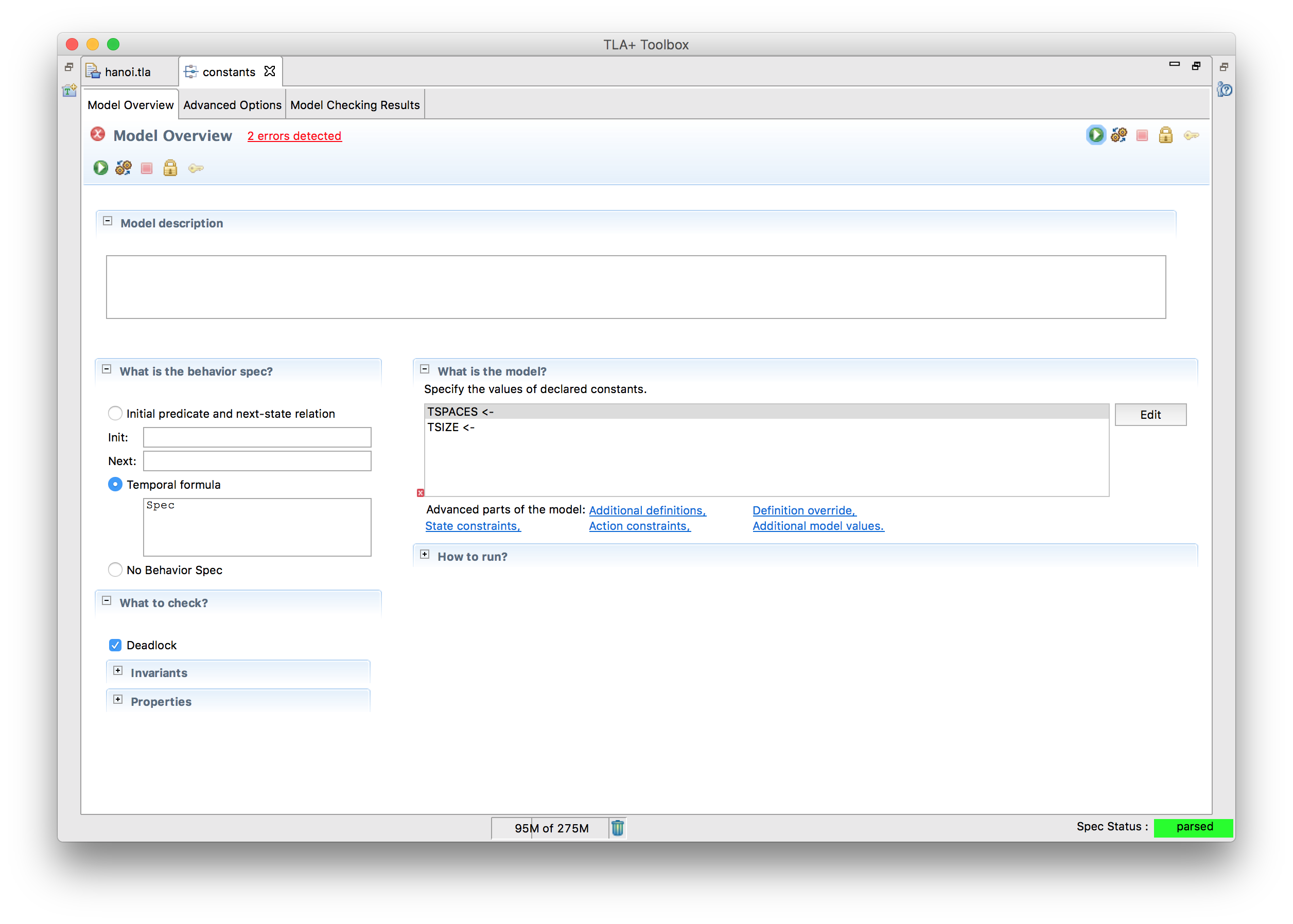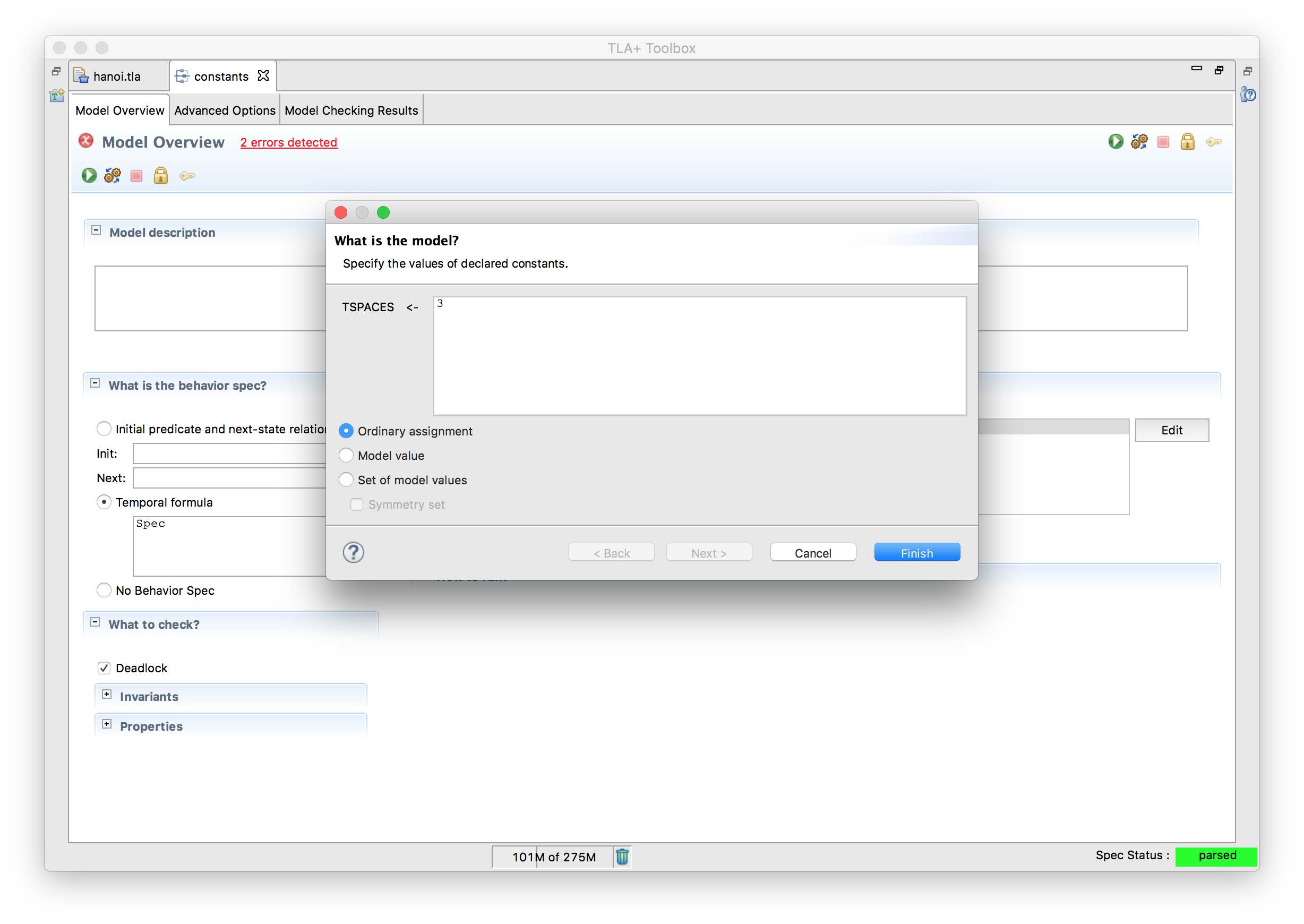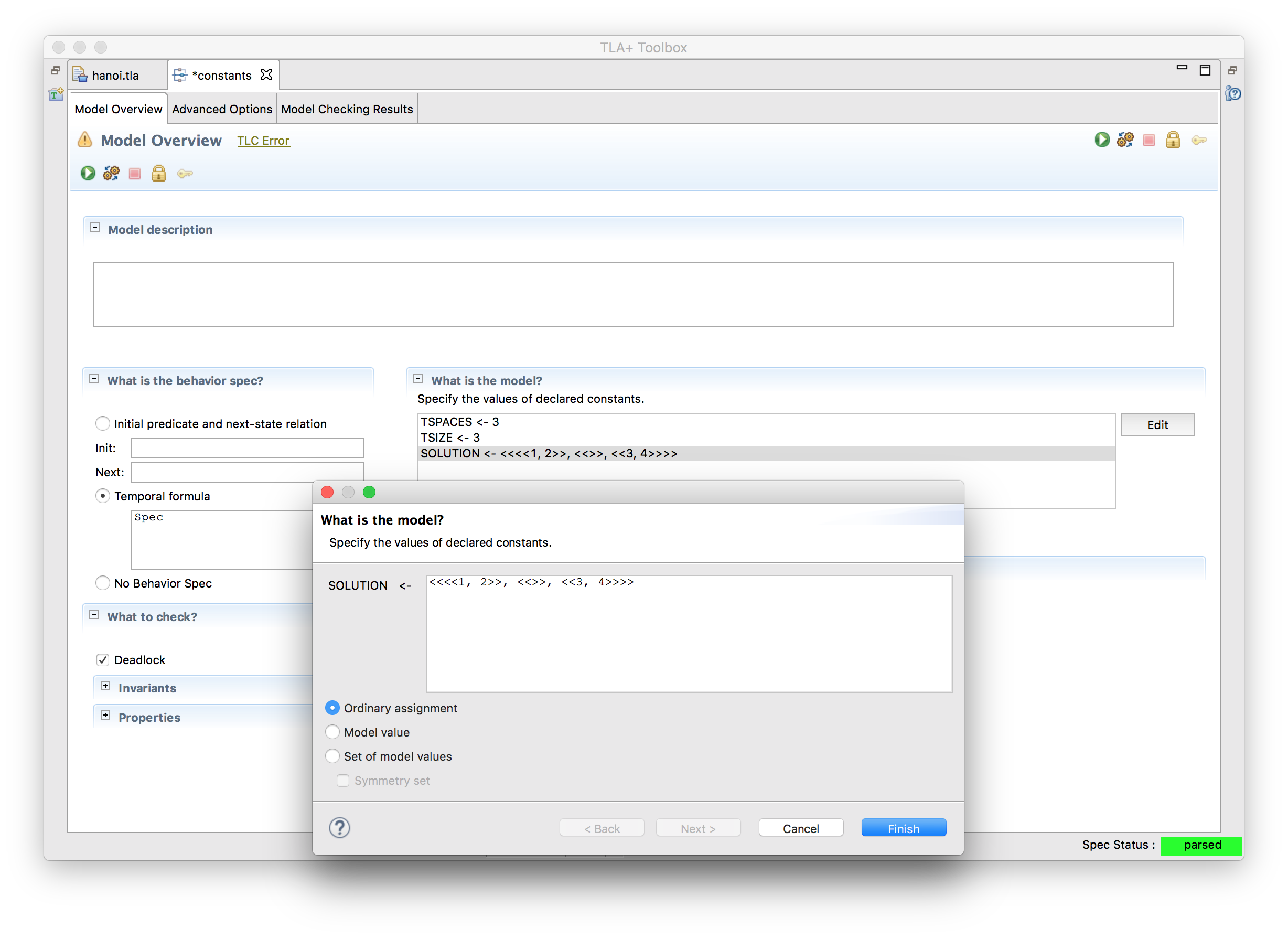# Constants

Let’s go back to our old Hanoi solver. As a refresher, this is the code we started with.

---- MODULE hanoi ----
EXTENDS TLC, Sequences, Integers

(* --algorithm hanoi
variables tower = <<<<1, 2, 3>>, <<>>, <<>>>>,

define
D == DOMAIN tower
end define;

begin
while TRUE do
assert tower /= <<1, 2, 3>>;
with from \in {x \in D : tower[x] /= <<>>},
to \in {
y \in D :
\/ tower[y] = <<>>
}
do
tower[from] := Tail(tower[from]) ||
end with;
end while;
end algorithm; *)
====


It works, but what if we wanted to change one of the parameters (more towers, more disks in a tower), we’d have to directly edit the code. It would be better to make a generic spec and test different parameters in the models instead. To do this, we introduce constants.

CONSTANTS TSIZE, TSPACES

FullTower[n \in 1..TSIZE] == n \* <<1, 2, 3, ...>>
Board[n \in 1..TSPACES] == IF n = 1 THEN FullTower ELSE <<>>

(* --algorithm hanoi
variables tower = Board;

define
D == DOMAIN tower
end define;

begin
while TRUE do
assert tower[TSPACES] /= FullTower;
\* rest is the same


This is the same as the old code, except that we now define the tower in terms of constants. Instead of the spec assigning the constant a value, the model does instead.When we click edit we can assign a specific value to each constant.### Assumptions

What if we also wanted to vary the solution? For example, we all know that it’s possible to move the entire tower over. But is it possible to reach <<<<1, 2>>, <<>>, <<3, 4>>>>? By moving the solution to a constant, we can vary that in the model, too:

CONSTANT SOLUTION
\* ...
assert tower /= SOLUTION


And now we can put the new solution in an ordinary assignment:One problem: this solution is nonsensical. It has four numbers when TSIZE is 3. In these cases, TLA+ can’t find a solution because the solution isn’t even defined! One way to catch this kind of error is with an ASSUME statement:

IsSensical(state) ==  /\ Len(state) = TSPACES \* Correct spaces
/\ \A tower \in DOMAIN state: \* Numbers do not exceed TSIZE
\A disc \in DOMAIN state[tower]:
state[tower][disc] \in 1..TSIZE
/\ \A n \in 1..TSIZE : \* All numbers appear
\E tower \in DOMAIN state:
\E disc \in DOMAIN state[tower]:
n = state[tower][disc]
\* ...

ASSUME IsSensical(SOLUTION)


TLC will check that our assignments don’t break any of the ASSUME expressions, so we can use ASSUME to make sure nobody makes a bad model. Of course, it can only check what we remember to check: for example, a solution with two “5”s can still slip through. As always, with programming, be paranoid.